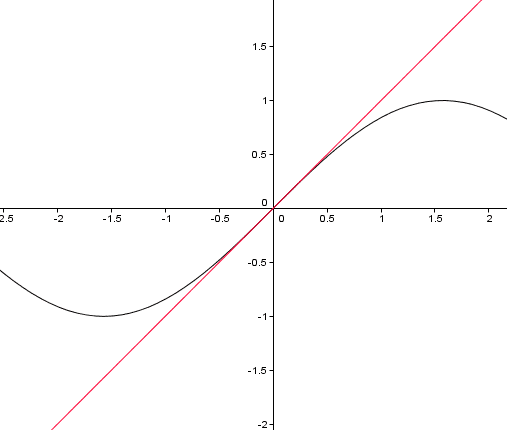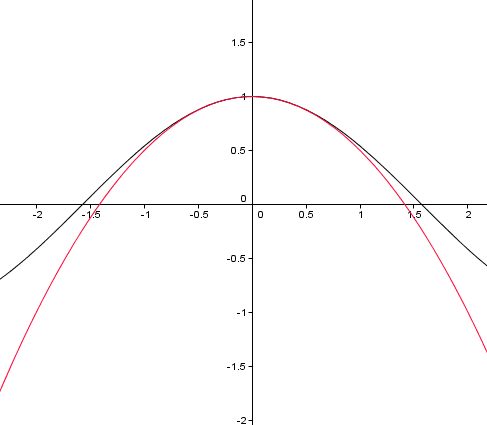# Explanation of small angle approximation?

< Mentor Note -- The OP's question was in the thread title. They have been advised to please make more detailed and clear thread starters >

thanks

Last edited by a moderator:

The most obvious way to explain these approximations is truncating the Taylor series about zero. If you haven't seen these, they're basically infinite series for the trig functions. In the case of sine and cosine, they are
$$\sin\theta = \sum_{n=0}^\infty \frac{(-1)^n \theta^{2n+1}}{(2n+1)!}\text{ and }\cos\theta = \sum_{n=0}^\infty \frac{(-1)^n \theta^{2n}}{(2n)!}$$
When $\theta\approx 0$, these approximations will get better and better for smaller numbers of terms. Where do they come from, you ask? Well, in many cases this is how mathematicians define sine and cosine. Otherwise it takes quite a bit of calculus to come up with these formulas, but you can check that they indeed work. Now, going back to $\theta\approx 0$, we write out the first term of sine and the second term of cosine (basically, we're taking terms with $\theta^2$ or lower exponents. We end up with, lo and behold
$$\sin\theta\approx\theta\text{ and }\cos\theta\approx 1-\frac{\theta^2}{2}.$$
Indeed, if you use a graphing program to graph this you get some very promising results around $\theta = 0$.The first image plots sine in black and $f(x)=x$ in red, the second image plots cosine in black and its approximation in red. Amazingly, the approximations don't look so bad!

Of course, there are always geometric explanations too, but this is the slightly more sophisticated way to view the approximations.

•berkeman
Where do they come from, you ask? Well, in many cases this is how mathematicians define sine and cosine. Otherwise it takes quite a bit of calculus to come up with these formulas,

I wouldn't say so - taking the derivative of sin(x) and cos(x) repeatedly and plugging into Taylor's equation is simple enough for anyone who asks this question!

Rationalising why Taylor's theorem works is slightly harder...

The most obvious way to explain these approximations is truncating the Taylor series about zero. If you haven't seen these, they're basically infinite series for the trig functions. In the case of sine and cosine, they are
$$\sin\theta = \sum_{n=0}^\infty \frac{(-1)^n \theta^{2n+1}}{(2n+1)!}\text{ and }\cos\theta = \sum_{n=0}^\infty \frac{(-1)^n \theta^{2n}}{(2n)!}$$
When $\theta\approx 0$, these approximations will get better and better for smaller numbers of terms. Where do they come from, you ask? Well, in many cases this is how mathematicians define sine and cosine. Otherwise it takes quite a bit of calculus to come up with these formulas, but you can check that they indeed work. Now, going back to $\theta\approx 0$, we write out the first term of sine and the second term of cosine (basically, we're taking terms with $\theta^2$ or lower exponents. We end up with, lo and behold
$$\sin\theta\approx\theta\text{ and }\cos\theta\approx 1-\frac{\theta^2}{2}.$$
Indeed, if you use a graphing program to graph this you get some very promising results around $\theta = 0$.

View attachment 76762

View attachment 76763

The first image plots sine in black and $f(x)=x$ in red, the second image plots cosine in black and its approximation in red. Amazingly, the approximations don't look so bad!

Of course, there are always geometric explanations too, but this is the slightly more sophisticated way to view the approximations.
thank you very much for that. I noticed when I tried to find the point of intersection of sinx and tanx graphically they were x = 0, pi, 2pi

how does this mean sinx = tanx ?? I thought it meant sinx only equals tan x when x is 0 pi or 2pi??
tah again

Fredrik
Staff Emeritus
Gold Member
how does this mean sinx = tanx ??
It doesn't, but when x is small, we have ##\cos x\approx1##, and therefore
$$\tan x=\frac{\sin x}{\cos x}\approx \frac{\sin x}{1}=\sin x.$$

Edit: I typed ##\cos x=1## above, but I meant ##\cos x\approx 1##. I fixed that after the reply below.

Last edited:
It doesn't, but when x is small, we have ##\cos x=1##, and therefore
$$\tan x=\frac{\sin x}{\cos x}\approx \frac{\sin x}{1}=\sin x.$$
ah thank you very much that makes sense :D

Fredrik
Staff Emeritus
Gold Member
Recall the definition of the derivative:
$$f'(x)=\lim_{h\to 0}\frac{f(x+h)-f(x)}{h}.$$ This implies that when ##h## is small, we have
$$f'(x)\approx \frac{f(x+h)-f(x)}{h}$$ for all ##x##, and therefore
$$f(x+h)\approx f(x)+f'(x)h$$ for all ##x##. This implies that when ##h## is small, we have
$$f(h)\approx f(0)+f'(0)h.$$ This result tells us in particular that when ##x## is small, we have
$$\sin x\approx\sin 0+\sin'(0)x =0+\cos 0\cdot x=x,$$ and
$$\cos x\approx\cos 0+\cos'(0)x= 1-\sin 0\cdot x =1.$$

It doesn't, but when x is small, we have ##\cos x\approx1##, and therefore
$$\tan x=\frac{\sin x}{\cos x}\approx \frac{\sin x}{1}=\sin x.$$

Edit: I typed ##\cos x=1## above, but I meant ##\cos x\approx 1##. I fixed that after the reply below.
thank very much :D but why does that result in sin x = x

sin(x) = x - x^3/3! + x^5/5! - x^7/7! + x^9/9! + ...
cos(x) = 1 - x^2/2! + x^4/4! - x^6/6! + x^8/8! + ...

For x<1, x^n<x(<1) for all n>1. For very small x, by extension, x^n is even smaller for all n>1; so for x close to 0, x^3 and x^5 and further is extremely close to 0 and can be called 0. So, for x close to 0,

sin(x) = x
cos(x) = 1

approximately.

(So tan(x) = sin(x)/cos(x) = sin(x)/1 (using cos(x)=1) = x/1 (using sin(x)=x) = x approximately, for x close to 0)

These relationships are all exactly true for x=0 (just as they are approximately true for x close to 0) and equivalently for x=2pi*n where n is any integer, because the trigonometric functions are periodic over 2pi (because 2pi is 360 degrees and the functions are defined geometrically, sin(x+2pi*n)=sin(x) etc.).

Fredrik
Staff Emeritus